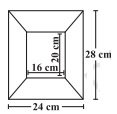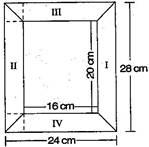# Diagram of the adjacent picture frame has outer dimensions = 24 cm×28 cm and inner dimensions 16 cm×20 cm. Find the area of each section of the frame, if the width of each section is same.Solution:

Divide the given figure into 4 parts, as shown below:Now two of given figures (I) and (II) are similar in dimensions.

Also figures (III) and (IV) are similar in dimensions.

Area of figure (I) = Area of trapezium

= (½) × (a + b) × h

= (½) × (28 + 20) × 4

= (½) × 48 × 4 = 96

Area of figure (I) = 96 cm2

Hence, Area of figure (II) = 96 cm2

Now, Area of figure (III) = Area of trapezium

= (½) × (a + b) × h

= (½) × (24 + 16)4

= (½) × 40 × 4 = 80

Area of figure (III) is 80 cm2

Hence, Area of figure (IV) = 80 cm2